# Introduction to Arrays – Data Structure and Algorithm Tutorials

## What is an Array?

An array is a collection of items of the same variable type that are stored at contiguous memory locations. It’s one of the most popular and simple data structures and is often used to implement other data structures. Each item in an array is indexed starting with 0.

The dream of every programmer is to become not just a good, but also a great programmer. We all want to achieve our goals and to achieve our goals, we must have a great plan with us. In this context, we have decided to provide a complete guide for Arrays interview preparation, which will help you to tackle the problems that are mostly asked in the interview, such as What is an Array, What is Array in C language, How do you initialize an Array in C, How to sort an Array, etc. We have also covered the topics such as Top Theoretical interview questions and Top interview coding questions in this complete guide for Array interview preparation.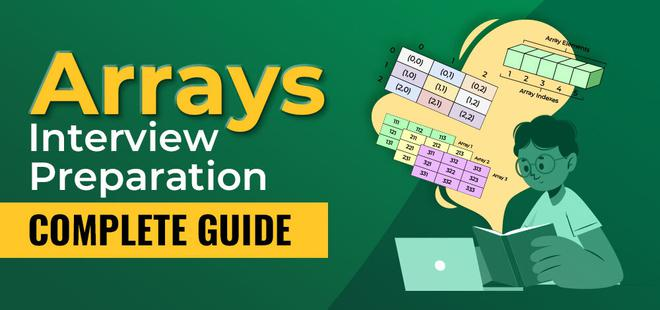Complete guide for Arrays interview preparation

We can directly access an array element by using its index value.

## Basic terminologies of array

• Array Index: In an array, elements are identified by their indexes. Array index starts from 0.
• Array element: Elements are items stored in an array and can be accessed by their index.
• Array Length: The length of an array is determined by the number of elements it can contain.

## Representation of Array

The representation of an array can be defined by its declaration. A declaration means allocating memory for an array of a given size.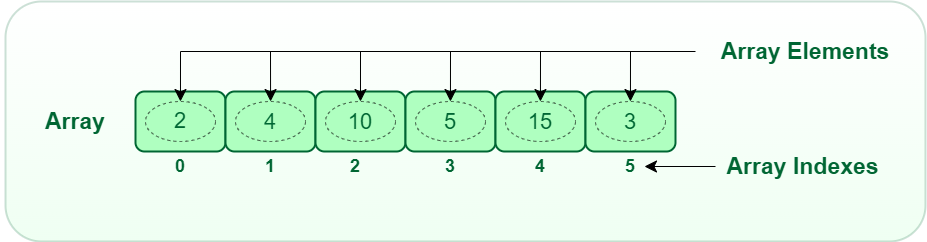Array

Arrays can be declared in various ways in different languages. For better illustration, below are some language-specific array declarations.

## C++

 `int` `arr;        ``// This array will store integer type element` `char` `arr;    ``// This array will store char type element` `float` `arr;  ``// This array will store float type element`

## C

 `int` `arr;        ``// This array will store integer type element` `char` `arr;    ``// This array will store char type element` `float` `arr;  ``// This array will store float type element`

## Java

 `/* Static Arrays are arrays that are declared before runtime` `and are assigned values while writing the code.` `*/`   `// The syntax of declaring a static array is:` `[]` `    ``= {, ,….. };`   `// Example:` `int` `arr[] = { ``2``, ``5``, ``6``, ``9``, ``7``, ``4``, ``3` `};`

## Python

 `# Python code` `arr ``=` `[``10``, ``20``, ``30``]  ``# This array will store integer` `arr2 ``=` `[``'c'``, ``'d'``, ``'e'``]  ``# This array will store characters` `arr3 ``=` `[``28.5``, ``36.5``, ``40.2``]  ``# This array will store floating elements`

## C#

 `int``[] arr = ``new` `int``; ``// This array will store integer type element`

## Javascript

 `// JS code` `let arr=[10,20,30];  ``// This array will store integer` `let arr2 = [``'c'``, ``'d'``, ``'e'``] ``// This array will store characters` `let arr3 = [28.5, 36.5, 40.2] ``// This array will store floating elements`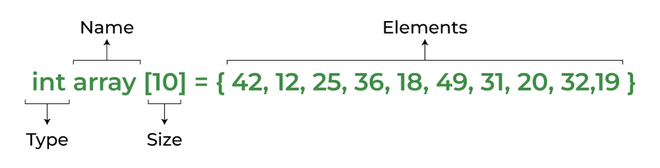Array declaration

However, the above declaration is static or compile-time memory allocation, which means that the array element’s memory is allocated when a program is compiled. Here only a fixed size (i,e. the size that is mentioned in square brackets []) of memory will be allocated for storage, but don’t you think it will not be the same situation as we know the size of the array every time, there might be a case where we don’t know the size of the array. If we declare a larger size and store a lesser number of elements will result in a wastage of memory or either be a case where we declare a lesser size then we won’t get enough memory to store the rest of the elements. In such cases, static memory allocation is not preferred.

### Is it possible to create dynamic array?

The answer is Yes. It is possible to allocate memory dynamically. So, dynamic memory allocation is the process of assigning the memory space during the execution time or the run time.

Below are the languages that support dynamic memory allocation:

## C++

 `int` `*array = ``new` `int``;`

## Java

 `// The syntax of declaring a dynamic array is:`   `// [ ];` `// Example: ` `int` `arr[``10``]; ``// Store integer elements` `String arr[``5``]; ``// Store String type of elements`

## Python3

 `# list of integers` `my_list ``=` `[``1``, ``2``, ``3``, ``4``]`   `# Empty list` `my_list ``=` `[]`   `# list of mixed data types` `my_list ``=` `[``"Hello"``, ``1``, ``5.5``]`

## C#

 `int``[] numArray = ``new` `int``[] {};`

## Javascript

 `// JavaScript has dynamic arrays: their size is not predetermined, nor the type of data.`   ` ``// Create array using literal` `var` `x = [``"a"``, ``"b"``, ``"c"``];   `   `// Create array using constructor` `var` `x = ``new` `Array();        `   `// Create array using constructor with items` `var` `y = ``new` `Array(``"a"``, ``"b"``, ``"c"``);`

## PHP

 `\$arr` `= ``array``(``"a"``,``"b"``,``"c"``);`

## Why Array Data Structures is needed?

Assume there is a class of five students and if we have to keep records of their marks in examination then, we can do this by declaring five variables individual and keeping track of records but what if the number of students becomes very large, it would be challenging to manipulate and maintain the data.

What it means is that, we can use normal variables (v1, v2, v3, ..) when we have a small number of objects. But if we want to store a large number of instances, it becomes difficult to manage them with normal variables. The idea of an array is to represent many instances in one variable..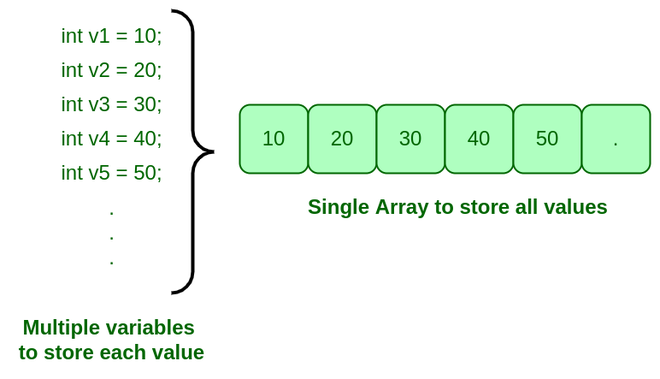Need for Array

## Types of arrays:

There are majorly two types of arrays: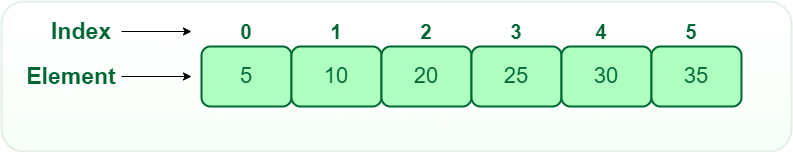1D array

• Two-dimensional array: 2-D Multidimensional arrays can be considered as an array of arrays or as a matrix consisting of rows and columns.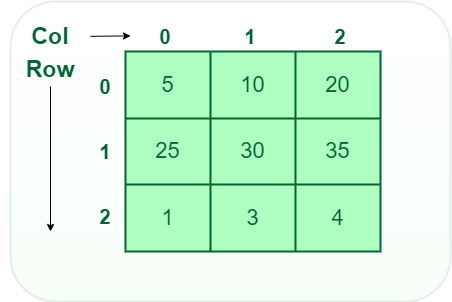2D array

• Three-dimensional array: A 3-D Multidimensional array contains three dimensions, so it can be considered an array of two-dimensional arrays.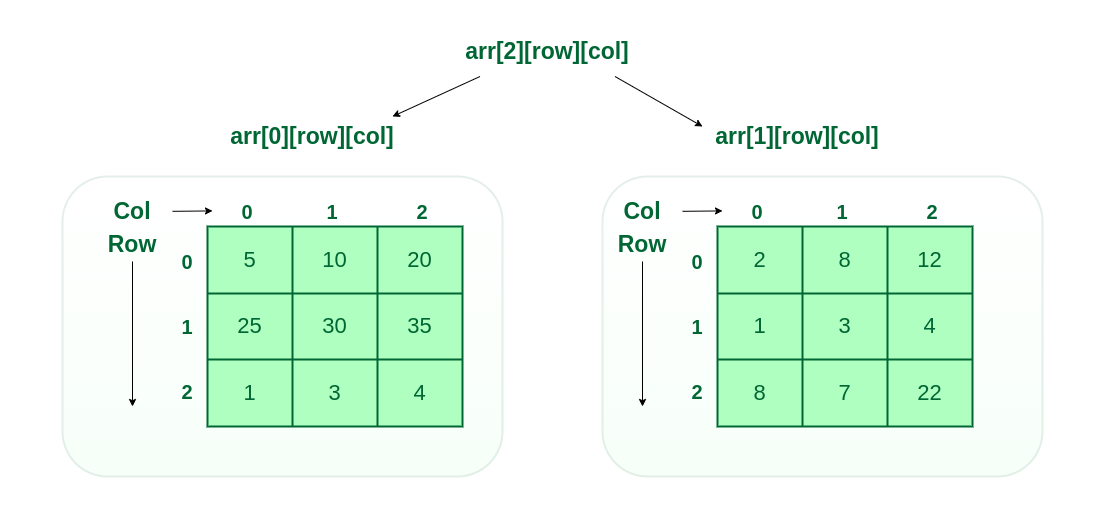3D array

## Types of Array operations:

• Traversal: Traverse through the elements of an array.
• Insertion: Inserting a new element in an array.
• Deletion: Deleting element from the array.
• Searching:  Search for an element in the array.
• Sorting: Maintaining the order of elements in the array.

• Arrays allow random access to elements. This makes accessing elements by position faster.
• Arrays have better cache locality which makes a pretty big difference in performance.
• Arrays represent multiple data items of the same type using a single name.
• Arrays store multiple data of similar types with the same name.
• Array data structures are used to implement the other data structures like linked lists, stacks, queues, trees, graphs, etc.

• As arrays have a fixed size, once the memory is allocated to them, it cannot be increased or decreased, making it impossible to store extra data if required. An array of fixed size is referred to as a static array.
• Allocating less memory than required to an array leads to loss of data.
An array is homogeneous in nature so, a single array cannot store values of different data types.
• Arrays store data in contiguous memory locations, which makes deletion and insertion very difficult to implement. This problem is overcome by implementing linked lists, which allow elements to be accessed sequentially.

## Application of Array:

• They are used in the implementation of other data structures such as array lists, heaps, hash tables, vectors, and matrices.
• Database records are usually implemented as arrays.
• It is used in lookup tables by computer.
• It is used for different sorting algorithms such as bubble sort insertion sort, merge sort, and quick sort.

## Top theoretical interview questions

#### Question

1 What will happen if you do not initialize an Array? View
2 Why is the complexity of fetching a value from an array be O(1) View
3 When should you use an Array over a List? View
4 What is a circularly sorted array? View
5 Comparing two arrays using hashmap? View
6 “What are the advantages of a linked list over an array? In which scenarios do
we use LinkedList and when Array?”
View
7 How do I iterate rows and columns of a multidimensional array? View
8 What is meant by Sparse Array? View
9 What are the benefits of Heap over Sorted Arrays?  View
10 Is there any difference between int[] a and int a[]? View
11 Can we declare array size as a negative number? View
12 We know that Arrays are objects so why cannot we write strArray.length()? View
13 What are the advantages of Sorted Arrays?   View
14 What defines the dimensionality of an Array?   View
15 How to check array contains a value or not? View
16 How to create an array/list inside another array/list? View
17 How to get the largest and smallest number in an array? View
18 How can I return coordinates/indexes of a string in a multidimensional array? View
19 How do I remove objects from an array in Java? View
20 How does C allocate data items in a multidimensional array? View
21 Get adjacent elements in a two-dimensional array? View
22 C++ How to use and pass a 3-dimensional char array? View
23 Anonymous Array in Java View
24  What is the default value of Array in Java? View
25 How to copy an array into another array? View
26 How to iterate an array in java? View
27 How to merge two sorted Arrays into a Sorted Array? View
28 Can we make the array volatile in Java? View
29 What is the logic to reverse the array? View
30 How to get the index of an array element? View
31 Can we extend an array after initialization? View
32 How to fill elements (initialize at once) in an array? View
33 Difference between Array and String in Java View
34 Print all subarrays with 0 sum View
35 Equilibrium index of an array View
36 How to check array contains a value or not? View
37 How to get the top two numbers from an array? View
38 How to implement 3 Stacks with one Array?  View

## Top 50 interview coding question

### Easy Problems on Arrays

#### Practice

1 Write a program to reverse the array View Solve
2 Find the minimum and maximum element in an array View Solve
3 Peak Element View Solve
4 Write a program to sort the given array View Solve
5 Find the Kth largest and Kth smallest number in an array View Solve
6 Find the occurrence of an integer in the array View Solve
7 Sort the array of 0s, 1s, and 2s View Solve
8 Subarray with given Sum View Solve
9 Move all the negative elements to one side of the array View Solve
10 Find the Union and Intersection of the two sorted arrays View Solve

### Medium Problems on Arrays

#### Practice

1 Write a program to cyclically rotate an array by one View Solve
2 Find the missing integer View Solve
3 Count Pairs with given sum View Solve
4 Find duplicates in an array View Solve
5 Sort an Array using the Quicksort algorithm View Solve
6 Find common elements in three sorted arrays View Solve
7 Find the first repeating element in an array of integers View Solve
8 Find the first non-repeating element in a given array of integers View Solve
9 Subarrays with equal 1s and 0s View Solve
10 Rearrange the array in alternating positive and negative items View Solve
11 Find if there is any subarray with a sum equal to zero View Solve
12 Find the Largest sum contiguous Subarray View Solve
13 Find the factorial of a large number View Solve
14 Find Maximum Product Subarray View Solve
15 Find the longest consecutive subsequence View Solve
16 Find the minimum element in a rotated and sorted array View Solve
17 Max sum in the configuration View Solve
18 Minimum Platforms View Solve
19 Minimize the maximum difference between the heights View Solve
20 Minimum number of jumps to reach the end View Solve
21 Stock Span problem View Solve
23 Find a triplet that sums to a given value View Solve
23 Smallest positive missing number View Solve
24 Find the row with a maximum number of 1’s View Solve
25 Print the matrix in a Spiral manner View Solve
26 Find whether an array is a subset of another array View Solve
27 Implement two Stacks in an array View Solve
28 Majority Element View Solve
29 Wave Array View Solve
30 Trapping Rainwater View Solve

### Hard Problems

#### Practice

1 Maximum Index View Solve
2 Max sum path in two arrays View Solve
3 Find Missing And Repeating View Solve
4 Stock buy and sell Problem View Solve
5 Pair with the given sum in a sorted array View Solve
6 Chocolate Distribution Problem View Solve
7 Partition Equal Subset Sum View Solve
8 Smallest Positive integer that can’t be represented as a sum View Solve
9 Coin Change Problem View Solve
10 Longest Alternating subsequence View Solve

### 1. What is an array in data structure with example?

An array is a collection of items of the same data type stored at contiguous memory locations. Ex. int arr = {1,2,3,4,5};

### 2. Why array is a data structure?

Arrays store elements of the same type, they are classified as homogeneous data structures. They can store numbers, strings, characters, boolean values (true and false), objects, and so on.

### 3. What data structure is an array?

An array is a linear data structure that stores similar elements in contiguous memory locations.

### 4. What are the types of arrays?

There are majorly two types of arrays:

• One dimensional array
• Multidimensional array

### 5. How is data stored in an array?

An array is a collection of items of the same data type stored at contiguous memory locations or says the elements are stored one after another in memory. An array uses an index system starting at 0 and going to (n-1), where n is its size.

### 6. Difference between array and structure?

The structure can contain variables of different types but an array only contains variables of the same type.

### 7. What are the limitations of an array?

An array is a collection of items of the same data type. That means, in an integer array only integer values can be stored, while in a float array only floating values and character array can have only characters. Thus, no array can have values of two data types.

### 8. What are the advantages of an array?

There are multiple advantages of array data structure and some of them are:

• Arrays allow random access to elements. This makes accessing elements by position faster.
• Arrays store multiple data of similar types with the same name.
• Array data structures are used to implement the other data structures like linked lists, stacks, queues, trees, graphs, etc.

### 9. What is the purpose of using arrays?

An array is used when several variables of the same type need to be used, and it can be defined as a sequence of objects of the same type.

### 10. What is a multidimensional array?

A multi-dimensional array can be termed as an array of arrays that stores homogeneous data in tabular form. Data in Multidimensional Arrays are stored in row-major order.

## Conclusion

After the discussion, we concluded that arrays are a simple method of accessing elements of the same type by grouping them and we can find the elements efficiently by their indexes and can perform different operations using them. Thus, they are more efficient when it comes to memory allocation and should be used in all modern programming languages. So, this becomes a favorite topic for the perspective of the interview and most of the companies generally asked about the problems on the array. For all these reasons, we must have a good knowledge of it.

Related articles:

Feeling lost in the world of random DSA topics, wasting time without progress? It's time for a change! Join our DSA course, where we'll guide you on an exciting journey to master DSA efficiently and on schedule.
Ready to dive in? Explore our Free Demo Content and join our DSA course, trusted by over 100,000 geeks!

Previous
Next